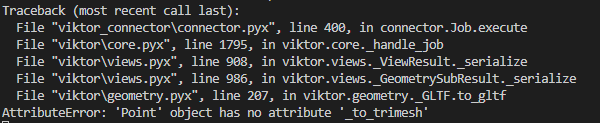# 2D visualization

Hi team,
what is the best way to visualize a point or a line or a polyline in a view?
I am creating a line or a point but I have this problem.``````    @GeometryView("LineView", duration_guess=1, view_mode='2D')
def line_vis(self, params, **kwargs):
pt1 = vg.Point(0, 0, 0)
pt2 = vg.Point(10, 10, 10)
line = vg.Line(pt1, pt2)
return GeometryResult(line)
``````

Don’t tell me I have to use Plotly or create an SVG fileHi, the GeometryView currently only supports `TransformableObject`s or a GLTF file. I would advice to use the latter, which you can easily create in trimesh (see Topics - GeometryView | VIKTOR Documentation). A line can then be visualized using a Path3D.

Thanks, @khameeteman
could please show me an example?
And what about points using trimesh?
I am asking for an example because I never used trimesh and they have a different why of building elements, I have to provide entities…
So an example could help

An example is provided in this section: Topics - GeometryView | VIKTOR Documentation.

Points can be created using a PointCloud

Yeah, I saw that example and it is clear.
I’m trying to understand the requirements for a Path2d and Path3d trimesh.path — trimesh 3.12.6 documentation
what are entities and how I should pass the vertices?
This is what I asked for an example.

I understand, it is not as straightforward and I noticed that you will also need `shapely` and `scipy` as separate requirements while they are not actually necessary for this part of the trimesh code. So I can imagine that simply passing a viktor line should be much easier. I will bump our internal issue.

Anyway, this should work:

``````
@GeometryView(...)
def geometry_view(self, **kwargs):
import trimesh

p1 = [0, 0, 0]
p2 = [1, 1, 0]

line = trimesh.path.entities.Line(points=[0, 1])
path = trimesh.path.Path3D(entities=[line], vertices=[p1, p2])
scene = trimesh.Scene(geometry=path)
geometry = File()
with geometry.open_binary() as w:
w.write(trimesh.exchange.gltf.export_glb(scene))

return GeometryResult(geometry)
``````
1 Like